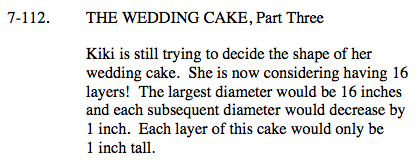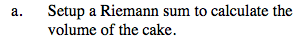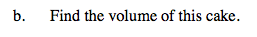### Home > CALC > Chapter 7 > Lesson 7.3.1 > Problem7-112

7-112.Since there are 16 layers of cake, and 16 is a large number, it would be beneficial to write the Riemann sum in sigma notation:

Δx represents the height of each cylindrical layer of cake.

Figure out the radius of the smallest layer. This will give you the initial value a.No need to expand. Use a calculator to evaluate the sigma notation.